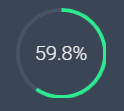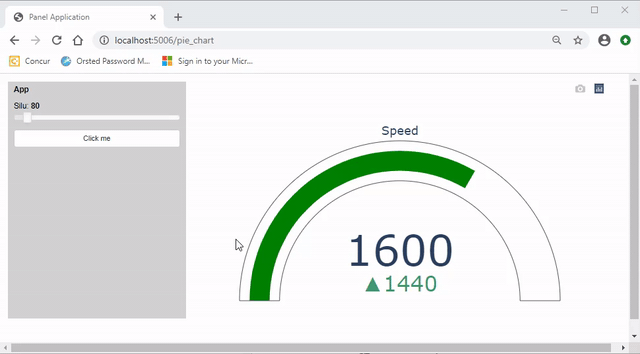# How to create a semi-pie chart with percentage?

Is it possible to create something like below with the holoviz libraries?1 Like

I don’t think so. I would really like something like this as well.

There is a Feature Request here https://github.com/holoviz/holoviews/issues/4363. I’ve added your request there.

Feel free to like it to get it higher on the priority list.

2 Likes

But you can use Plotly in Panel and Plotly provides these things here https://plotly.com/python/indicator/

And if you don’t mind using `panel.pane.HTML` you can use html and css to create it. See https://codepen.io/vineethtrv/pen/xGjQOX

1 Like

Thanks again for your insightful solutions!

1 Like

If you get something working and can share a screenshot and/ or code, then please share. Would be awesome to see. Thanks.

Hello !!! I tried to use a plotly indicator to indicate some properties in my panel. I update the plotly figure with pn.depends but the new figure overlaps with the old figure. You can see the gif

I do similar things with matplotlib figure and the old figure disappears with the same approach. The MRE is the next one:

``````
import time
import panel as pn
pn.extension("plotly")

import plotly.graph_objects as go

fig = go.Figure()

slider = pn.widgets.IntSlider(name='silu', start =0, end = 1000, value = 0, width = 200)

@pn.depends(slider.param.value)
def fip(e):
value = 20*slider.value,
delta = {'reference': 160},
gauge = { 'axis': {'visible': False} },
domain = {'row': 0, 'column': 0}
)
)

fig.update_layout(
grid = {'rows': 1, 'columns': 1, 'pattern': "independent"},
template = {'data' : {'indicator': [{
'title': {'text': "Speed"},
'mode' : "number+delta+gauge",
'delta' : {'reference': 90}}]
}})
return fig

def b(e):
for i  in range (10):
slider.value += 10
print ('value changed')
time.sleep(1)

btn = pn.widgets.Button(name = 'button', width =200)

btn.on_click(b)

pn.Row(pn.Column(btn, slider), fip).show()

``````

I tried to run this code with .show in the notebook and bith bokeh serve in the terminal, but the behaviour is the same.

Thanks for the help,
N

You are almost there. The problem is that you append more and more traces. You need to clear the data of the figure each time you run fip: `fig.data = []`

``````import time
import panel as pn
pn.extension("plotly")

import plotly.graph_objects as go

fig = go.Figure()

slider = pn.widgets.IntSlider(name='silu', start =0, end = 1000, value = 0, width = 200)

@pn.depends(slider.param.value)
def fip(e):
fig.data = []
value = 20*slider.value,
delta = {'reference': 160},
gauge = { 'axis': {'visible': False} },
domain = {'row': 0, 'column': 0}
)
)

fig.update_layout(
grid = {'rows': 1, 'columns': 1, 'pattern': "independent"},
template = {'data' : {'indicator': [{
'title': {'text': "Speed"},
'mode' : "number+delta+gauge",
'delta' : {'reference': 90}}]
}})
return fig

def b(e):
for i  in range (10):
slider.value += 10
print ('value changed')
time.sleep(1)

btn = pn.widgets.Button(name = 'button', width =200)

btn.on_click(b)

pn.Row(pn.Column(btn, slider), fip).show(port=5007)
``````

Thank you very much for the quick response @Marc !!!

I noticed a glitch when the semi-pie chart updates. Its because you put `fip` in as a function. It’s “slow” as it needs to determine the type of object and transfer a Plotly pane to the browser for each update. If you define a Plotly pane instead and just update the `.object` it works better.

I’ve created a parametrized example below. I just love the Parameterized apiI tried to optimize for efficiency as described here https://panel.holoviz.org/reference/panes/Plotly.html but it is not totally clear to me if I did the “perfect” thing.

``````import time
import panel as pn
import param

pn.extension("plotly")

import plotly.graph_objects as go

class App(param.Parameterized):
silu = param.Integer(default=0, bounds=(0, 1000))
click_me = param.Action()
view = param.Parameter()

def __init__(self, **params):
super().__init__(**params)

self.settings_pane = pn.Param(
self,
parameters=["silu", "click_me"],
sizing_mode="fixed",
height=400,
width=300,
background="lightgrey",
)
self.fig = self._get_figure()
self.figure_pane = pn.pane.Plotly(self.fig, sizing_mode="stretch_width")
self.view = pn.Column(
pn.Row(self.settings_pane, self.figure_pane, sizing_mode="stretch_both"),
sizing_mode="stretch_both",
)

self.click_me = self._click_me
self._update_figure()

def _get_figure(self):
fig = go.Figure()
fig.update_layout(
grid={"rows": 1, "columns": 1, "pattern": "independent"},
template={
"data": {
"indicator": [
{
"title": {"text": "Speed"},
"mode": "number+delta+gauge",
"delta": {"reference": 90},
}
]
}
},
)
return fig

@param.depends("silu", watch=True)
def _update_figure(self):
self.fig.data=[]
go.Indicator(
value=20 * self.silu,
delta={"reference": 160},
gauge={"axis": {"visible": False}},
domain={"row": 0, "column": 0},
)
)
self.figure_pane.object = self.fig.to_dict()

def _click_me(self, events):
for i in range(10):
if self.silu + 10 < 1000:
self.silu += 10
else:
self.silu = 0
print("value changed")
time.sleep(0.33)

App().view.servable()
``````Thank you very much again !!! I noticed the glitch several times in many scripts and I thought it was normal. With your explanation and the object replacement suggestion I could solve the glicth in a lot of differents scripts.

Thank for the explanation and awesome-panel !!!

1 Like

I continue exploring gauges, Now I embedded the echarts library in a HTML pane to obtain another gauge.

``````import time
import panel as pn
import param

pn.extension("plotly")

import plotly.graph_objects as go

pn.config.js_files["echart1"] = "https://cdn.bootcss.com/echarts/3.7.2/echarts.min.js"

pn.config.sizing_mode = "stretch_width"

STYLE = """
body {
margin: 0px;
min_height: 100vh;
}
.bk.app-bar {
background: #212121;
border-color: white;
color: #ffffff;
z-index: 50;
}

"""

pn.config.raw_css.append(STYLE)

top_bar = pn.Row(pn.pane.Markdown("# Classic Dashboard in Panel ", margin=(10, 5, 10, 25)),
pn.Spacer(height=0),
pn.pane.PNG(
"https://panel.holoviz.org/_static/logo_horizontal.png",
width=200,
sizing_mode="fixed",
align="center",
margin=(10, 50, 10, 5),
),
css_classes=["app-bar"],
)

### slider to control the value of the gauges  ###

int_slider = pn.widgets.IntSlider(name='Integer Slider', start=0, end=100, step=1, value=4, width=200)

# initialization of plotly pane

fig = go.Figure()
value = 2,
delta = {'reference': 1},
gauge = { 'axis': {'visible': False} },
domain = {'row': 0, 'column': 0}
)
)
fig.update_layout(
grid = {'rows': 1, 'columns': 1, 'pattern': "independent"},
template = {'data' : {'indicator': [{
'title': {'text': "Speed"},
'mode' : "number+delta+gauge",
'delta' : {'reference': 45}}]
}})
plotly_pane = pn.pane.Plotly(fig)

### initialization of echarts gauge ###

html = """
<div id="855be12876564e2fb3fd5fe122d3d221" class="chart-container" style="width:500px; height:300px;"></div>
"""

script = """
<script>
var myScript = document.currentScript;
var myDiv = myScript.parentElement.firstElementChild;
var myChart = echarts.init(myDiv);
myDiv.eChart = myChart;

var option = {
tooltip: {
formatter: '{a} <br/>{b} : {c}%'
},
toolbox: {
feature: {
restore: {},
saveAsImage: {}
}
},
series: [
{
name: 'Echarts velocimeter',
type: 'gauge',
detail: {formatter: '{value}%'},
data: [{value: 50, name: 'vel'}]
}
]
};

option.series.data.value = 25;
myChart.setOption(option, true);

myDiv.after_layout = myChart.resize; // Resizes the chart after layout of parent element

</script>

"""

ech = pn.pane.HTML(html+script, margin=15)

### Here I create a dummy HTML pane to send commands to update the echarts gauge ###
ech_dummy = pn.pane.HTML("")

@pn.depends(int_slider.param.value, watch=True)
def update_gauges(e):
# update plotly pane
fig.data = []
value = int_slider.value ,
delta = {'reference': 2},
gauge = { 'axis': {'visible': False} },
domain = {'row': 0, 'column': 0}
)
)
fig.update_layout(
grid = {'rows': 1, 'columns': 1, 'pattern': "independent"},
template = {'data' : {'indicator': [{
'title': {'text': "Speed"},
'mode' : "number+delta+gauge",
'delta' : {'reference': 45}}]
}})

plotly_pane.object = fig.to_dict()

# update echarts HTML pane
part1 = " <script> option.series.data.value = "
part3 = " ; myChart.setOption(option, true); </script> "
ech_dummy.object = part1 + str(int_slider.value) + part3

pn.Column(top_bar,
pn.Row(int_slider,
plotly_pane,
ech),
ech_dummy).show()
``````

Here you have a screenshot of the gauges working

Thank you very much to the panel developers,
it’s an amazing library to present my scientific results !!!

1 Like